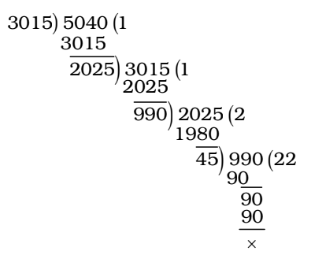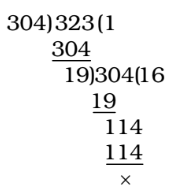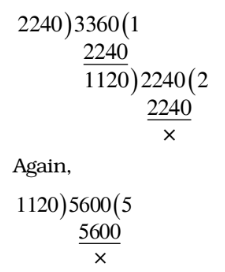## LCM and HCF

#### LCM and HCF

1. Which greatest number will divide 3026 and 5053 leaving remainders 11 and 13 respectively?

1. We know that the largest number which when divide the numbers a, b and c give remainders as p, q, r respectively is given by H.C.F. of (a – p), (b – q) and (c – r).
3026 –11 = 3015 and 5053 –13 = 5040
Required number = HCF of 3015 and 5040##### Correct Option: C

We know that the largest number which when divide the numbers a, b and c give remainders as p, q, r respectively is given by H.C.F. of (a – p), (b – q) and (c – r).
3026 –11 = 3015 and 5053 –13 = 5040
Required number = HCF of 3015 and 5040∴ Required number = HCF of 3015 and 5040 = 45

1. What is the greatest number that will divide 307 and 330 leaving remainders 3 and 7 respectively ?

1. As we know that the largest number which when divide the numbers a, b and c give remainders as p, q, r respectively is given by H.C.F. of (a – p), (b – q) and (c – r).
The number will be HCF of 307 – 3 = 304 and 330 – 7 = 323.##### Correct Option: A

As we know that the largest number which when divide the numbers a, b and c give remainders as p, q, r respectively is given by H.C.F. of (a – p), (b – q) and (c – r).
The number will be HCF of 307 – 3 = 304 and 330 – 7 = 323.∴ Required number = 19

1. Let N be the greatest number that will divide 1305, 4665 and 6905 leaving the same remainder in each case. Then, sum of the digits in N is :

1. We can say that the largest number which when divide the numbers a, b and c give remainders as p, q, r respectively is given by H.C.F. of (a – p), (b – q) and (c – r).
The greatest number N = HCF of (1305 – t ), (4665 – t ) and (6905 – t), where t is the remainder
= HCF of (4665 – 1305), (6905– 4665) and (6905 – 1305)
= HCF of 3360, 2240 and 5600##### Correct Option: A

We can say that the largest number which when divide the numbers a, b and c give remainders as p, q, r respectively is given by H.C.F. of (a – p), (b – q) and (c – r).
The greatest number N = HCF of (1305 – t ), (4665 – t ) and (6905 – t), where t is the remainder
= HCF of (4665 – 1305), (6905– 4665) and (6905 – 1305)
= HCF of 3360, 2240 and 5600∴ N = 1120
Sum of digits = 1 + 1 + 2 + 0 = 4

1.  H.C.F of 2 , 4 and 6 is 3 5 7

1.  H.C.F of 2 , 4 and 6 3 5 7

As we know that ,
 H.C.F. of fractions = HCF of numerators LCM of denominators

##### Correct Option: B

 H.C.F of 2 , 4 and 6 3 5 7

As we know that ,
 H.C.F. of fractions = HCF of numerators LCM of denominators

 H.C.F. = HCF of 2, 4 and 6 LCM of 3, 5 and 7

 H.C.F. = 2 105

1. The greatest number, which when divide 989 and 1327 leave remainders 5 and 7 respectively, is :

1. We know that The largest number which when divide the numbers a, b and c give remainders as p, q, r respectively is given by H.C.F. of (a – p), (b – q) and (c – r).
Required number = HCF of (989 – 5) and (1327 – 7)
Required number = HCF of 984 and 1320

##### Correct Option: C

We know that The largest number which when divide the numbers a, b and c give remainders as p, q, r respectively is given by H.C.F. of (a – p), (b – q) and (c – r).
Required number = HCF of (989 – 5) and (1327 – 7)
Required number = HCF of 984 and 1320 = 24
∴ HCF = 24